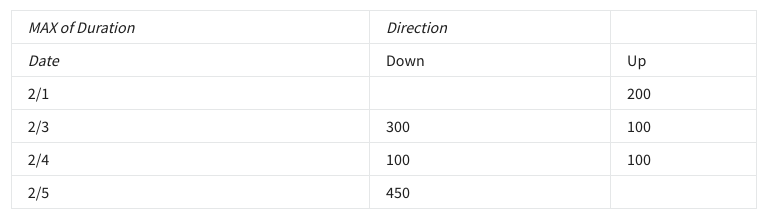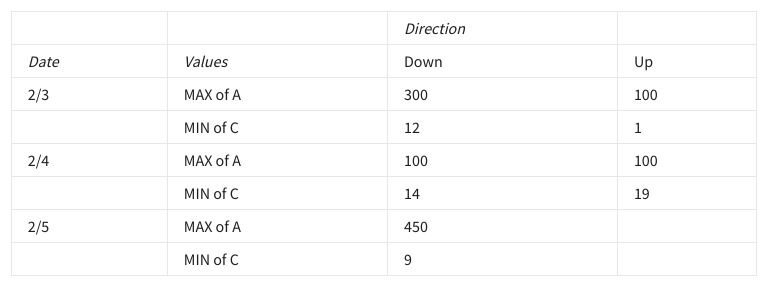## Week 4 – Performing data calculations – Shuffle Q/A 1

• AVERAGE
• SUM
• PRODUCT
• COUNTA

#### 12. What column is set as a value in the following pivot table?• Direction
• Duration
• MAX
• Date

#### 13. In the following SQL query, which column is part of an addition operation that creates a new column?SELECT Yes_Responses, No_Responses, Total_Surveys, Yes_Responses + No_Responses AS Responses_Per_SurveyFROM Survey_1

• Total_Surveys
• Responses_Per_Survey
• Yes_Responses
• Survey_1

• /
• !=
• <>
• %

#### 15. What is the purpose of using data validation during your analysis process?

• To ensure that you are able to use every piece of data from your raw data
• To guarantee that all of your stakeholders will be happy with your results
• To ensure that all data is complete, accurate, secure, and consistent
• To guarantee that visualizations are visually pleasing

#### 16. What is the purpose of the <> operator in SQL?

• To return the remainder of a division operation
• To check if two values are not equal
• To set a value equal to another

#### 17. What is a reason to use a temporary table instead of a standard table in SQL?

• A temporary table allows functions that are unavailable to standard tables.
• A temporary table calculates formulas using less memory than standard tables.
• A temporary table calculates formulas faster than standard tables.
• A temporary table allows analysts to repeatedly work with the same subset of data.

#### 18. Which of the following SQL queries adds a table into the database?

• SELECT * FROM table GROUP BY columnA ORDER BY columnB;
• CREATE TABLE my_table AS (SELECT * FROM other_table);
• SELECT * FROM table;
• WITH my_table AS (SELECT * FROM other_table WHERE x = 0);

## Shuffle Q/A 2

#### 19. What is the purpose of using pivot tables?

• To multiply two arrays and add the results
• To allow quick copying from one table to another
• To view data in multiple ways to find insights and trends
• To allow the use of SQL in spreadsheets

#### 20. How many different columns have been added to the values section of the pivot table editor?• 3
• 2
• 6
• 1